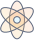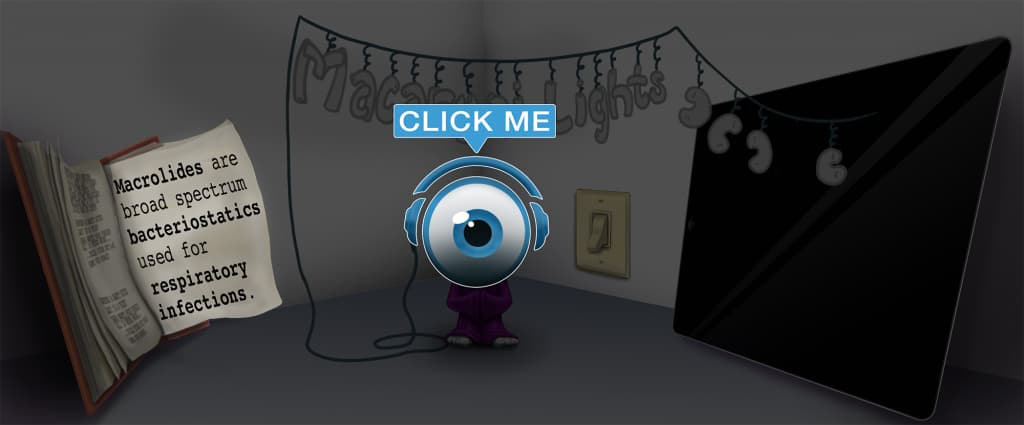# Master Capacitance with Picmonic for College

## With Picmonic, facts become pictures. We've taken what the science shows - image mnemonics work - but we've boosted the effectiveness by building and associating memorable characters, interesting audio stories, and built-in quizzing.

### Capacitance

CapsPicmonic

Capacitors are circuit components used to temporarily store charge. They are similar to batteries, but batteries store charge for longer periods and discharge at a slower rate. The most commonly tested capacitors are parallel plate capacitors. They exist in two phases: charging and discharging.

A capacitor charges when it is hooked up to a direct current battery, which supplies electrons to the circuit. Electrons flow from the negative terminal of the battery to one of the capacitor plates, and the capacitor stores charge. That plate becomes the negative plate of the capacitor, and the electron buildup creates an electric field that repels electrons from the other plate. The other plate then becomes the positive plate.

Once charged, the capacitor can be hooked up to a circuit without a battery and with a device needing power. The capacitor releases charge into the circuit, and electrons flow from the negative plate through a connecting wire to the positive plate. If a light bulb was also connected to that wire, it would be powered by the current flowing from the negative plate of the capacitor.

There are two equations for capacitance. The first is C = Q / V. The Capacitance (Farads) of the capacitor is equal to the Charge (Coulombs) stored on the capacitor divided by the Voltage (Volts) of the Battery. As either Capacitance or Voltage increase, the Charge stored also increases.

The second equation is C = ε0(A/d), and applies to parallel plate capacitors. A is the area of the plates, and d is the distance between the plates. As area increases, the capacitance increases and the capacitor stores more charge. As the distance between the plates increases, the capacitance decreases and the capacitor stores less charge.

11 KEY FACTS
Charging
Electron yelling "CHARGE!" and Charging into Caps

When a capacitor is hooked up to a circuit with a battery, it stores charge from the current released by the battery.

Direct Current (DC) Battery Supplies Charge
DC Battery Supplying Electrons

The most common way of charging a capacitor is by connecting a direct current battery to the same circuit. The DC battery supplies current, or electrons, to the circuit.

Electrons Flow to Negative Plate
Electrons Flowing to Negative Plate

When the DC battery is hooked up to the circuit, electrons flow from the negative terminal of the battery to one of the capacitor plates. That capacitor plate becomes negatively charged. It is important to note that conventionally, when we talk about "current flow" in a circuit, it flows from the positive terminal of the battery to the negative terminal. However, that was before scientists determined that electrons were the charged carriers. Thus, while "current", denoted as an I, flows from positive to negative, in reality electrons flow from negative to positive.

Capacitor Stores Charge
Electrons stored in Caps

The capacitor stores up charge on the negative plate because electrons build up from the current flowing out of the battery.

Electric Field from Negative Plate Repels Electrons on Positive Plate
Electron Repels Electron on Positive Plate with Lightning

The electric field created by the charge on the negative plate repels electrons away from the other plate, creating a relative positive plate. Both plates have equal absolute values of charge, but one is negative while the other is positive.

Discharging
Dice yelling "DISCHARGE!" and discharging from Caps

Once charged, a capacitor can be hooked up to a circuit without a battery and act as the source of current for the circuit. In the second circuit, the capacitor discharges.

Capacitor releases charge
Capacitor releasing Electrons onto Circuit

The capacitor releases charge, or electrons, from the negative plate into the circuit.

Electrons Flow from Negative to Positive Plate
Electrons Flowing from Negative Plate around to Positive Plate

Electrons always flow from negative terminal to positive terminal. In the case of a capacitor discharging, electrons flow from the negative capacitor plate, where electrons were stored during the charging phase, to the positive plate. The two plates are connected by a wire.

Light Bulb is Powered by Capacitor
Light Bulb Powered by Electrons from Caps

In a circuit with a discharging capacitor, there is usually a circuit component that is powered by the current flowing from the capacitor. It is often a light bulb, which is powered by the electrons flowing from the capacitor's negative plate.

C = Q / V
C-Cap EQUALS Q-Lamb OVER V-Vaulter

The fundamental equation of capacitance is C = Q/V. C is capacitance, measured in Farads. Q is charge, measured in Coulombs. V is voltage, measured in Volts. One way to think of the equation is that when a capacitor of capacitance "C" is hooked up to a battery with voltage "V", you can calculate the amount of charge, "Q", that builds up on the capacitor. There are also some relationships from the equation. As Voltage (V) of the battery increases and Capacitance (C) remains the same, the Charge (Q) also increases. If the Capacitance (C) is increased, the charge (Q) is increased with constant Voltage (V).

C = ε0(A/d)
C-Cap EQUALS Epsilon-O beside A-Arrow OVER D-Ruler

The most commonly tested capacitors are parallel plate capacitors. Two plates of area "A" are separated by a certain distance, "d". ε0 is a constant called the permittivity of free space, and does not need to be memorized. As the area of the plates increases (assuming constant distance), capacitance increases. A larger plate can store more charge. As the distance between the plates increases (assuming constant area), capacitance decreases. As the plates get farther apart, the positive plate experiences a weaker electric field from the negative plate and the "capacitance" decreases (meaning it stores less charge).

Unlock all 11 facts & definitions with Picmonic Free!

JOIN FREE

## Take the Capacitance Quiz

Picmonic's rapid review multiple-choice quiz allows you to assess your knowledge.

START QUIZ NOW

## Picmonic for College CoversCollege
300+ Videos
2,000+ Facts

## Our Story Mnemonics Increase Mastery and Retention

Memorize facts with phonetic mnemonics

Unforgettable characters with concise but impactful videos (2-4 min each)

### Ace Your College Classes & Exams with Picmonic:

#### Over 1,050,000 students use Picmonic’s picture mnemonics to improve knowledge, retention, and exam performance.